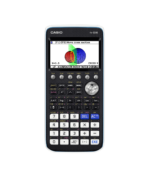Quick Skills Archives - Casio Calculators

# Quick Skills

Displaying Resources

FilterHow to Video
fx-CG50 A-Level GCSE IB
Quick Skill: Calculations Using Coordinate Values

How to extract coordinate values from points of interest in Graph to perform ope...How to Video
fx-CG50 A-Level GCSE IB
Quick Skill: Solving Equations with more than 1 Variable

How to use Solver to solve equations with more than one variable including SUVAT...How to Video
fx-CG50 IB A-Level GCSE
Quick Skill: Graphing – Storing and Recalling Functions

How to use Graph Memory to store functions and settings for later recall. You wi...How to Video
fx-CG50 Further Maths IB
Quick Skill: Matrices – Inverses and Powers

How to find the inverse of a matrix and raise a matrix to a power. You will need...How to Video
fx-CG50 IB A-Level GCSE
Quick Skill: Solving Linear Equations

How to solve a linear equation using the numerical SolveN function....How to Video
fx-CG50 A-Level GCSE IB
Quick Skill: Sequences – Generating Terms

How to generate a list of terms of a sequence based on a function and then sum t...How to Video
fx-CG50 A-Level IB
Quick Skill: Sequences – Summation

How to use sigma summation of the terms of a sequence....How to Video
fx-CG50 GCSE IB A-Level
Quick Skill: Table – Extracting Values

How to extract either a single column or a complete set of table values. You wil...How to Video
fx-CG50 A-Level GCSE IB
Quick Skill: Graphing – Drawing a Graph and Table

How to draw the graph of a function and create a table of values from the graph....How to Video
fx-CG50 A-Level GCSE IB
Quick Skill: Graphing – Drawing 2 Graphs

How to draw adjacent graphs for comparing functions. You will need to know how t...How to Video
fx-CG50 Further Maths
Quick Skill: Poisson and Geometric Distributions – Finding X-Values

How to calculate X-values and critical values when using the Distribution app. Y...How to Video
fx-CG50 Further Maths
Quick Skill: Geometric and Poisson Distributions – Probabilities

How to calculate Geometric and Poisson probabilities. You will need to know how ...How to Video
fx-CG50 A-Level IB
Quick Skill: Normal Distribution – Extracting X-Values

How to extract X-values calculated using the Distribution app. You will need to ...How to Video
fx-CG50 A-Level IB
Quick Skill: Probability Distributions – Using Probability Results

How to extract probabilities calculated using the Distribution app. You will nee...How to Video
fx-CG50 A-Level GCSE IB
Quick Skill: Quartiles – Using Summary Values

How to extract the quartile values of single-variable data found using the Stati...How to Video
fx-CG50 A-Level GCSE IB
Quick Skill: Statistics – Using Summary Values

How to extract the summary statistic values of single-variable data found using ...How to Video
fx-CG50 A-Level GCSE IB
Quick Skill: Graphs – Quick Scale Settings

How to use Zoom to automatically fill the graph with the sketched function, zoom...How to Video
fx-CG50 IB A-Level
Quick Skill: Graphing – Inverse Trigonometric Functions

How to graph the inverse trigonometric functions. Restricting the domain ensures...How to Video
fx-CG50 A-Level IB
Quick Skill: Graphing – Restricting the Domain of a Function

How to restrict the domain of a function when graphing. You will need to know ho...How to Video
fx-CG50 A-Level IB
Quick Skill: Calculations Using Function Notation

How to use function notation to perform operations in the Run-Matrix app. You wi...